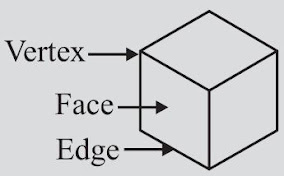# Chapter 15 Visualising Solid Shapes Class 7 Notes Maths

Here you will find Chapter 15 Visualising Solid Shapes Class 7 Notes Maths through which a student can boost their preparation and assessment of understood concepts. In Revision Notes for Class 7, information are arranged in precise manner that will make learning easier and more understandable. It is quite easy to retain the answers once you are fully aware of the concept thus notes can be beneficial for you. NCERT Solutions for Class 7 Chapter 15 Maths are prepared as per the accordance of latest CBSE guidelines so you can score maximum marks.Objects having different shapes (dimensions)

One Dimensional Objects

• Objects having length only. For example, line.

Two Dimensional Objects

• Figures having both length and breadth. For example, Rectangle, triangle etc. These figures can be easily represented on a plane. So, they are called plane figures. Plane figures are of two dimensions (2-D) so, they are also called 2-dimensional figure.

Three Dimensional Objects

• Objects and shapes having length, breadth and height. Solid figures are of three dimensions (3-D).

Faces, Vertices and Edges of solid shapes

• The flat surfaces of a solid figure are called its faces. The top and bottom faces are called bases.

• The line segments where the adjacent faces of a solid meet are called edges.

• The point where the edges of a solid intersect is called vertex.Nets for building 3D shapes

• A net is a skeleton-outline of a solid that can be folded to make the solid.

Drawing Solids on a Flat Surface

• Solid shapes can be drawn on a flat surface. This is called a 2-D representation of 3-D solid shape.

Two types of sketches of a solid are possible:
(i) An oblique sketch does not have proportional lengths. Still it conveys all important aspects of the appearance of the solid.
(ii) An isometric sketch is drawn on an isometric dot paper, a sample of which is given at the end of this book. In an isometric sketch of the solid the measurements kept proportional.

Different sections of a solid can be viewed in many ways:
(i) One way is to view by cutting or slicing the shape, which would result in the cross-section of the solid.
(ii) Another way is by observing a 2-D shadow of a 3-D shape.
(iii) A third way is to look at the shape from different angles; the front-view, the side-view and the top-view can provide a lot of information about the shape observed.